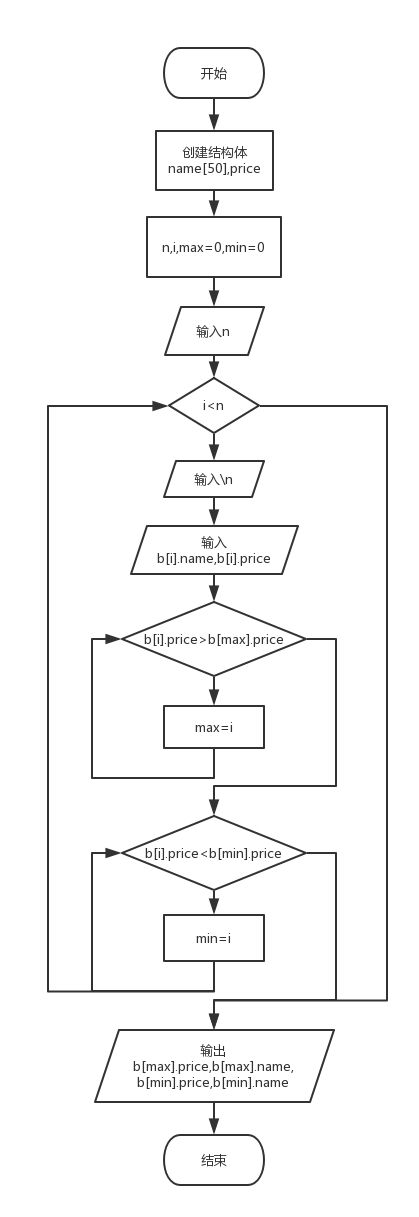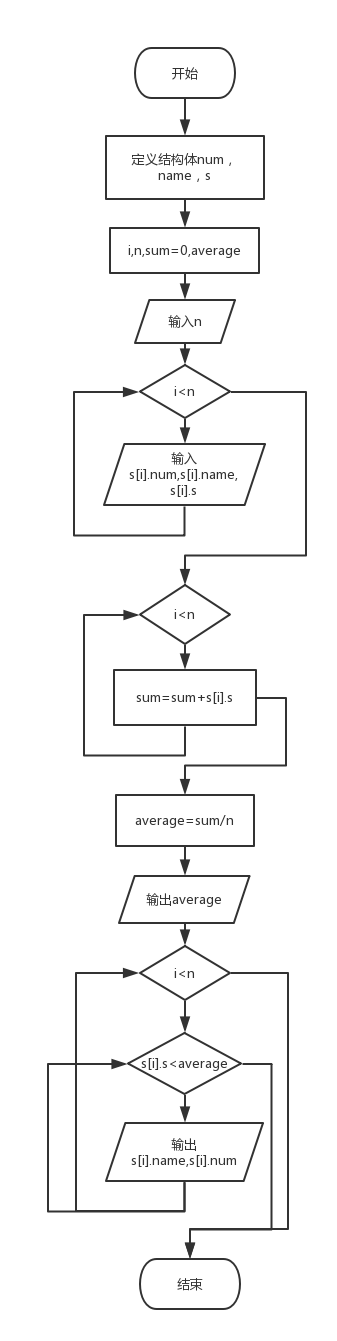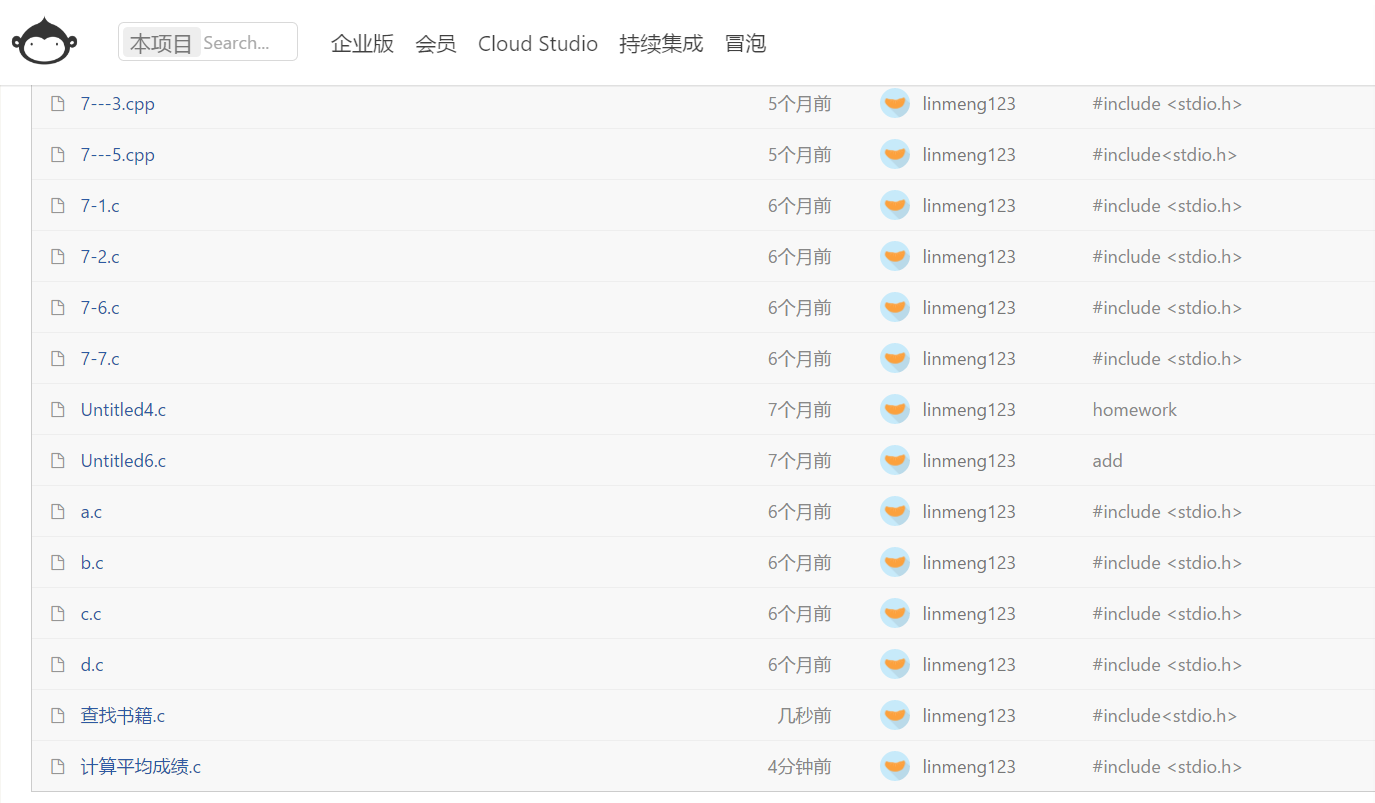## 第三次作业

1 设计思路
(1)描述题目算法

(2)流程图2.实验代码

#include<stdio.h>
#include<string.h>
#include<stdlib.h>
struct book
{
char name;
double cost;
} s;
main()
{
int i,n;
scanf("%d",&n);
for(i=0;i<n;i++)
{
scanf("\n");
gets(s[i].name);
scanf("%lf",&s[i].cost);
}
int max=0,min=0;
for(i=1;i<n;i++){
if(s[i].cost>s[max].cost){max=i;}
if(s[i].cost<s[min].cost){min=i;}
}
printf("%.2f, %s\n",s[max].cost,s[max].name);
printf("%.2f, %s",s[min].cost,s[min].name);
system("pause");
}

3.本题调试过程1 设计思路
(1)描述题目算法

(2)流程图2.实验代码

#include <stdio.h>
#include <stdlib.h>
#include <string.h>
struct score
{
char num;
char name;
int score;
};
int main()
{
int i,n,count=0;
float average=0;
scanf("%d",&n);
struct score s[n];
for(i=0;i<n;i++)
scanf("%s %s %d",&s[i].num,s[i].name,&s[i].score);
for(i=0;i<n;i++)
{
count+= s[i].score;
}
average = count / n;
printf("%.2f\n",average);
for(i = 0; i < n; i++)
{
if(s[i].score < average)
{
printf("%s %s\n",s[i].name,s[i].num);

}
}
system("pause");
}

3.本题调试过程1、指针与结构作业中题目所使用的知识点

2、源代码使用git提交posted on 2018-04-22 14:29  linmenga5  阅读(136)  评论(0编辑  收藏  举报